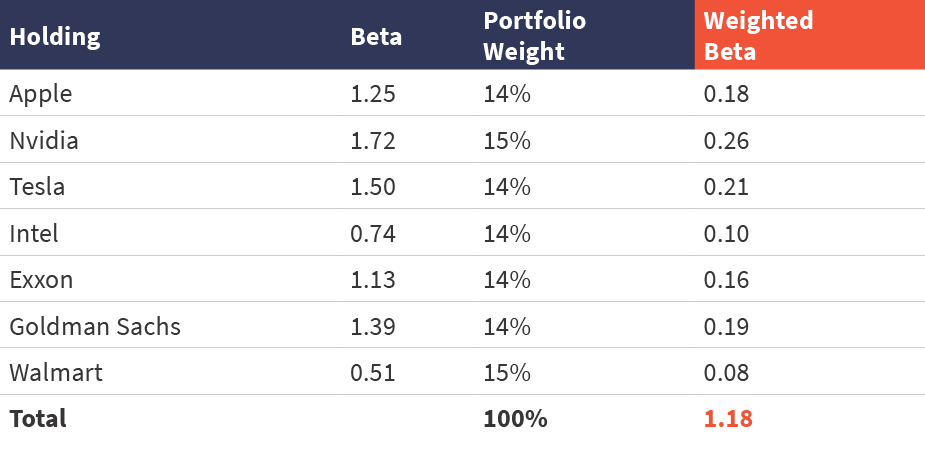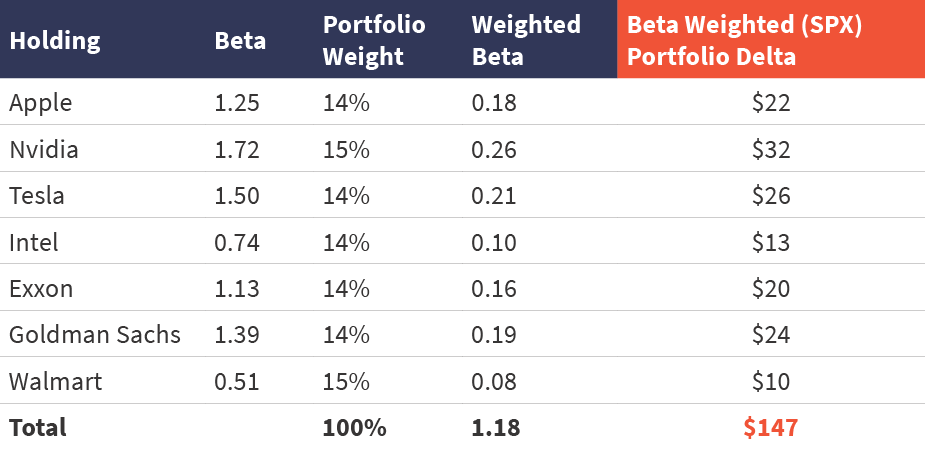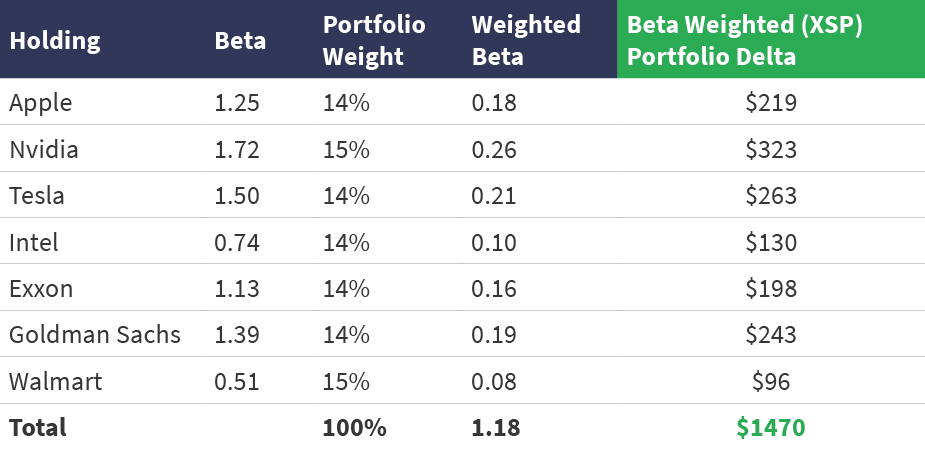# How to Right-size Hedges Via Beta Weighting with XSP Options

Guest Author
December 2, 2022

Guest Authors: Derek Moore and Jay Pestrichelli, ZEGA Financial

To hedge a portfolio of individual stocks, notional value alone may not tell the whole story.

Index options are a good way to protect your investments, but how much should you use? Will they be enough? The challenge is understanding the relationship between how much your positions will move for every corresponding move in the S&P 500® Index (SPX™).

The Cboe Mini-SPX Index options contract, known by its symbol XSP, allows right-sizing given its lower notional values.

## How to Calculate the Beta Weight of a Stock Portfolio

Beta represents how much an asset, in this case a stock, moves relative to the market SPX Index.

The market’s beta would equal 1 since that is the benchmark. If a stock is expected to move greater than the market, its beta would be greater than 1, while a stock expected to be less volatile than the market would have a beta of less than 1.

Within a portfolio of stocks, we can take each of their individual betas multiplied by the percentage weight within that portfolio to find the weighted beta.

Below, we created a hypothetical \$500,000 portfolio with seven holdings, including Nvidia (NVDA).

Since NVDA represents 15% of the portfolio, its weighted beta is equal to 0.26, calculated as (1.72 * .15 = 0.26).

The same process is repeated for each holding, and we find the sum of each result in the portfolio having a total weighted beta of 1.18.

## \$500,000 Hypothetical Portfolio Beta Weighted to SPX Priced at 4000.00Data Source: Thinkorswim

## How To Calculate the Beta Weighted Delta of a Stock Portfolio

The beta weighted delta informs us how much in dollars a portfolio will move up or down against a one point move by the referenced asset (SPX Index).

What’s the difference between the delta on an individual stock and the beta weighted delta?

• Individual stock delta explains how much an option on that stock will move compared to a 1-point move in that stock itself.
• Beta weighted delta shows how much a stock will make or lose for every 1-point move in the referenced asset.

Investment managers develop portfolio hedges using index options as the vehicle. Using an SPX Index level of 4000.00, a 1-point move represents a change of 0.025% (1/4000 = 0.00025). In our example of a \$500,000 portfolio, we would take [ (500,000 * .00025) * 1.18] = 147.5. When the market moves by 1 point (converted to percent change), that change is then multiplied by the portfolio weighted beta of 1.18.

The next 1-point change in the SPX Index is expected to result in a change of \$147.50.

## \$500,000 Hypothetical Beta Weighed Delta to SPX Priced at 4000.00Data Source: Thinkorswim

Let’s look at NVDA to see how this works.

Nvidia represents 15% of the portfolio. So (500,000 * .15) = 75,000. Since the referenced asset (SPX Index) moved 0.025%, to calculate its individual beta weighted delta, we take [(75,000 * .00025) * 1.72 = 32].

The beta weighted portfolio delta provides portfolio managers with a more refined view of the current risk when designing hedges.

## Notional Value Is Not Enough

Since options represent control but not ownership, contracts equate to a theoretical “notional” value.

Standard option contracts represent 100 shares of the underlying symbol. The simple way to figure it out is to multiply the number of contracts by underlying price by deliverable. For example:

• 1 Put Option on the SPX at 4000 represents \$400,000 notional (1 x 100 x 4000).

When designing hedges, notional values fall short offsetting delta. Purchasing an SPX at-the-money (ATM) put option with a delta around 50 would have the same notional value as buying an out-of-the-money (OTM) put with a 10 delta.

While these have the same notional exposure, they provide very different price changes related to market movement. Each will go up in value more for every 1-point move in the market but using the beta weighted delta lets managers size hedges based upon how much downside delta they want to protect.

## Benefit of Smaller Notional Size of XSP Mini-SPX Options

While SPX options are a powerful tool for hedging long portfolio exposure, the challenge is the higher notional size.

Want to hedge a portfolio worth \$550,000? One contract is too little, while two is too many.

This is where the flexibility of XSP options (based on the Mini-SPX Index, which is 1/10th the size of standard SPX Index) comes into play.

• 1 Put Option on the XSP at 400 represents \$40,000 notional (100 x 400).

Now let’s repeat the same process but this time insert the XSP Mini-SPX index as our referenced asset instead.

## XSP Mini-SPX Beta Weighted \$500,000 Portfolio with SPX at 4000.00Data Source: Thinkorswim

## Designing XSP Mini-SPX Hedge

With XSP, for every 10 points the SPX Index moves, the XSP 1/10th Index moves 1 point.

SPX Index = 4000.00    10 SPX points (1 XSP Point) = 0.0025% move in XSP.

Now we can see the impact of each 1-point move in the portfolio.

With a portfolio long 1470 beta weighted deltas, the portfolio manager can decide what percentage of that exposure they wish to hedge.

Let’s use the following XSP put in our example:

Long XSP May 2023 (178 Days to Exp) 360 Put @ \$10.40 Delta -24.

If we buy 12 of the above, we will control a notional \$480,000 on our \$500,000 portfolio. Since each contract is short -24 deltas, 12 contracts would represent -288 deltas total.

Should the SPX Index go down 10 points, 1 point lower for XSP, instead of the portfolio dropping \$1470, it would only drop (\$1470 - \$288) = \$1182, due to the short delta offset inherent in the long XSP puts.

Put another way, on the next 10-point drop in the SPX Index (1 XSP Point), you reduce the loss by 20%.

The cost to purchase this protection is \$12,480 or about a 5% annualized cost on the \$500,000 portfolio.

## Using Put Spreads to Reduce Cost and Harvest Volatility

Using an XSP put spread allows managers to reduce the cost and sell lower probability options below the market.

Long XSP May 2023 (178 Days to Exp) 360 Put @ \$10.40 Delta -24

Short XSP May 2023 (178 Days to Exp) 300 Put @ \$3.10 Delta   7

_______________________________________________________________________

Net \$7.30 Delta -17

Now you are short of a total of -204 deltas, so a 1-point drop in XSP would be offset (\$1470 - \$204 = \$1266). Here you are reducing the loss by about 14%. The annualized cost now falls to 3.5%. For the same amount of money buying just the long put, you could increase the number of contracts to 17 from 12.

In this structure, you would feel the first -10% down, and be protected from -10% until -25% in the market. Why? At expiration, the 360 put strike is 10% below the current 400 XSP price, while the 300 strike is 25% below.

Of course, between now and expiration, managers will provide a hedge with the potential for your deltas to increase along the way.

Picking a lower strike to sell premium at reduces your cost. Most managers probably would agree they don’t need a portfolio to be hedged all the way down to zero.

## Remember to Right-size

Now you can understand your relative risk to the market for a basket of stock positions.

Notional value is a good starting point but using beta weighted delta allows you to right-size your hedges based on what you want to protect, the amount you want to spend and how far down you need to hedge.